Home Practice
For learners and parents For teachers and schools
Textbooks
Full catalogue
Pricing SupportLog in

We think you are located in United States. Is this correct?

# 17.5 Parallel resistors

## 17.5 Parallel resistors (ESAFK)

When we add resistors in parallel to a circuit:

• There are more paths for current to flow which ensures that the current splits across the different paths.

• The voltage is the same across the resistors. The voltage across the battery in the circuit is equal to the voltage across each of the parallel resistors:

${V}_{\text{battery}} = {V}_{1} = {V}_{2} = {V}_{3} \ldots$
• The resistance to the flow of current decreases. The total resistance, $${R}_{P}$$, is given by:

$\frac{1}{{R}_{P}} = \frac{1}{{R}_{1}} + \frac{1}{{R}_{2}} + \ldots$

When resistors are connected in parallel the start and end points for all the resistors are the same. These points have the same potential energy and so the potential difference between them is the same no matter what is put in between them. You can have one, two or many resistors between the two points, the potential difference will not change. You can ignore whatever components are between two points in a circuit when calculating the difference between the two points.

Look at the following circuit diagrams. The battery is the same in all cases, all that changes is more resistors are added between the points marked by the black dots. If we were to measure the potential difference between the two dots in these circuits we would get the same answer for all three cases.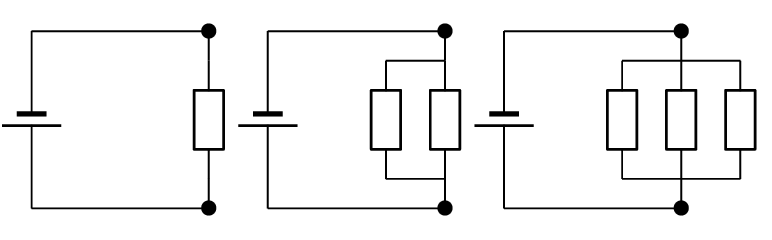Lets look at two resistors in parallel more closely. When you construct a circuit you use wires and you might think that measuring the voltage in different places on the wires will make a difference. This is not true. The potential difference or voltage measurement will only be different if you measure a different set of components. All points on the wires that have no circuit components between them will give you the same measurements.

All three of the measurements shown in the picture below (i.e. A–B, C–D and E–F) will give you the same voltage. The different measurement points on the left have no components between them so there is no change in potential energy. Exactly the same applies to the different points on the right. When you measure the potential difference between the points on the left and right you will get the same answer.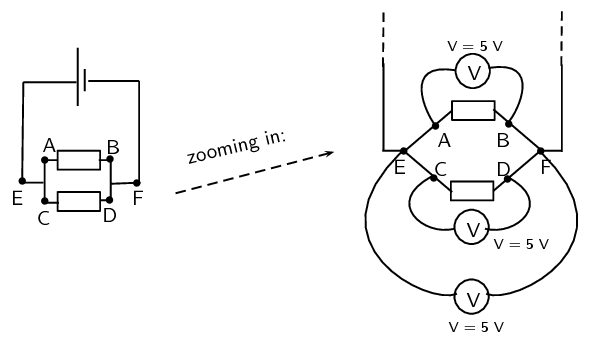## Worked example 7: Voltages I

Consider this circuit diagram: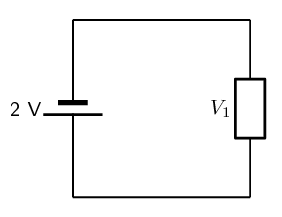What is the voltage across the resistor in the circuit shown?

### Check what you have and the units

We have a circuit with a battery and one resistor. We know the voltage across the battery. We want to find that voltage across the resistor.

${V}_{\text{battery}} = \text{2}\text{ V}$

### Applicable principles

We know that the voltage across the battery must be equal to the total voltage across all other circuit components.

${V}_{\text{battery}}={V}_{\text{total}}$

There is only one other circuit component, the resistor.

${V}_{\text{total}}={V}_{1}$

This means that the voltage across the battery is the same as the voltage across the resistor.

\begin{align*} {V}_{\text{battery}} & = {V}_{\text{total}} = {V}_{1} \\ {V}_{1} & = \text{2}\text{ V} \end{align*}

## Worked example 8: Voltages II

Consider this circuit: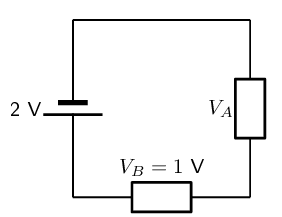What is the voltage across the unknown resistor in the circuit shown?

### Check what you have and the units

We have a circuit with a battery and two resistors. We know the voltage across the battery and one of the resistors. We want to find that voltage across the resistor.

\begin{align*} {V}_{\text{battery}} & = \text{2}\text{ V} \\ {V}_{B} & = \text{1}\text{ V} \end{align*}

### Applicable principles

We know that the voltage across the battery must be equal to the total voltage across all other circuit components that are in series.

${V}_{\text{battery}} = {V}_{\text{total}}$

The total voltage in the circuit is the sum of the voltages across the individual resistors

${V}_{\text{total}} = {V}_{A} + {V}_{B}$

Using the relationship between the voltage across the battery and total voltage across the resistors

\begin{align*} {V}_{\text{battery}} & = {V}_{\text{total}} \\\\ {V}_{\text{battery}} & = {V}_{1} + {V}_{\text{resistor}} \\ \text{2}\text{ V} & = {V}_{1} + \text{1}\text{ V} \\ {V}_{1} & = \text{1}\text{ V} \end{align*}

## Worked example 9: Voltages III

Consider the circuit diagram: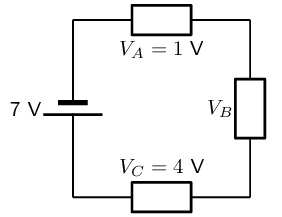What is the voltage across the unknown resistor in the circuit shown?

### Check what you have and the units

We have a circuit with a battery and three resistors. We know the voltage across the battery and two of the resistors. We want to find that voltage across the unknown resistor.

\begin{align*} {V}_{\text{battery}} & = \text{7}\text{ V} \\ {V}_{\text{known}} & = {V}_{A} + {V}_{C} \\ & = \text{1}\text{ V} + \text{4}\text{ V} \end{align*}

### Applicable principles

We know that the voltage across the battery must be equal to the total voltage across all other circuit components that are in series.

${V}_{\text{battery}}={V}_{\text{total}}$

The total voltage in the circuit is the sum of the voltages across the individual resistors

${V}_{\text{total}} = {V}_{B} + {V}_{\text{known}}$

Using the relationship between the voltage across the battery and total voltage across the resistors

\begin{align*} {V}_{\text{battery}} & = {V}_{\text{total}} \\ {V}_{\text{battery}} & = {V}_{B} + {V}_{\text{known}} \\ \text{7}\text{ V} & = {V}_{B} + \text{5}\text{ V} \\ {V}_{B} & = \text{2}\text{ V} \end{align*}

## Worked example 10: Voltages IV

Consider the circuit diagram: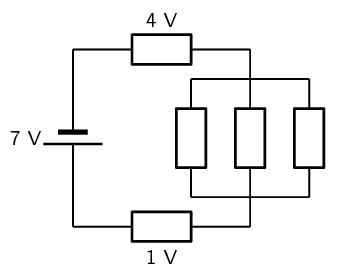What is the voltage across the parallel resistor combination in the circuit shown? Hint: the rest of the circuit is the same as the previous problem.

The circuit is the same as the previous example and we know that the voltage difference between two points in a circuit does not depend on what is between them so the answer is the same as above $${V}_{\text{parallel}} = \text{2}\text{ V}$$.

### Check what you have and the units - long answer

We have a circuit with a battery and five resistors (two in series and three in parallel). We know the voltage across the battery and two of the resistors. We want to find that voltage across the parallel resistors, $${V}_{\text{parallel}}$$.

\begin{align*} {V}_{\text{battery}} = \text{7}\text{ V} \\ {V}_{\text{known}} = \text{1}\text{ V} + \text{4}\text{ V} \end{align*}

### Applicable principles

We know that the voltage across the battery must be equal to the total voltage across all other circuit components.

${V}_{\text{battery}}={V}_{\text{total}}$

Voltages only add algebraically for components in series. The resistors in parallel can be thought of as a single component which is in series with the other components and then the voltages can be added.

${V}_{\text{total}}={V}_{\text{parallel}}+{V}_{\text{known}}$

Using the relationship between the voltage across the battery and total voltage across the resistors

\begin{align*} {V}_{\text{battery}} & = {V}_{\text{total}} \\ {V}_{\text{battery}} & = {V}_{\text{parallel}} + {V}_{\text{known}} \\ \text{7}\text{ V} & = {V}_{\text{parallel}} + \text{5}\text{ V} \\ {V}_{\text{parallel}} & = \text{2}\text{ V} \end{align*}
temp text

In contrast to the series case, when we add resistors in parallel, we create more paths along which current can flow. By doing this we decrease the total resistance of the circuit!

Take a look at the diagram below. On the left we have the same circuit as in the previous section with a battery and a resistor. The ammeter shows a current of $$\text{1}$$ $$\text{A}$$. On the right we have added a second resistor in parallel to the first resistor. This has increased the number of paths (branches) the charge can take through the circuit - the total resistance has decreased. You can see that the current in the circuit has increased. Also notice that the current in the different branches can be different.The total resistance of a number of parallel resistors is NOT the sum of the individual resistances as the overall resistance decreases with more paths for the current. The total resistance for parallel resistors is given by:

$\frac{1}{{R}_{P}}=\frac{1}{{R}_{1}}+\frac{1}{{R}_{2}}+\ldots$

Let us consider the case where we have two resistors in parallel and work out what the final resistance would be. This situation is shown in the diagram below: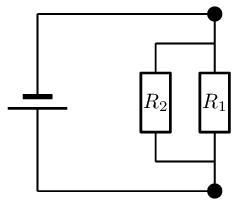Applying the formula for the total resistance we have:

\begin{align*} \frac{1}{{R}_{P}} & = \frac{1}{{R}_{1}} + \frac{1}{{R}_{2}} + \ldots \\ & \text{There are only two resistors} \\ \frac{1}{{R}_{P}} & = \frac{1}{{R}_{1}} + \frac{1}{{R}_{2}} \\ & \text{Add the fractions} \\ \frac{1}{{R}_{P}} & = \frac{1}{{R}_{1}} \times \frac{{R}_{2}}{{R}_{2}} + \frac{1}{{R}_{2}} \times \frac{{R}_{1}}{{R}_{1}} \\ \frac{1}{{R}_{P}} & = \frac{{R}_{2}}{{R}_{1}{R}_{2}} + \frac{{R}_{1}}{{R}_{1}{R}_{2}} \\ & \text{Rearrange} \\ \frac{1}{{R}_{P}} & = \frac{{R}_{2} + {R}_{1}}{{R}_{1}{R}_{2}} \\ \frac{1}{{R}_{P}} & = \frac{{R}_{1} + {R}_{2}}{{R}_{1}{R}_{2}} \\ {R}_{P} & = \frac{{R}_{1}{R}_{2}}{{R}_{1} + {R}_{2}} \end{align*}

For any two resistors in parallel we now know that

${R}_{P} = \frac{\text{product of resistances}}{\text{sum of resistances}} = \frac{{R}_{1}{R}_{2}}{{R}_{1} + {R}_{2}}$

## Current dividers

### Aim

Test what happens to the current and the voltage in series circuits when additional resistors are added.

• A battery

• A voltmeter

• An ammeter

• Wires

• Resistors

### Method

• Connect each circuit shown below

• Measure the voltage across each resistor in the circuit.

• Measure the current before and after each resistor in the circuit and before and after the parallel branches.### Results and conclusions

• Compare the currents through individual resistors with each other.

• Compare the sum of the currents through individual resistors with the current before the parallel branches.

• Compare the various voltage measurements across the parallel resistors.

## Worked example 11: Parallel resistors I

A circuit contains two resistors in parallel. The resistors have resistance values of $$\text{15}$$ $$\text{Ω}$$ and $$\text{7}$$ $$\text{Ω}$$.What is the total resistance in the circuit?

### Analyse the question

We are told that the resistors in the circuit are in parallel circuit and that we need to calculate the total resistance. The values of the two resistors have been given in the correct units, Ω.

### Apply the relevant principles

The total resistance for resistors in parallel has been shown to be the product of the resistances divided by the sum. We can use

${R}_{P} = \frac{{R}_{1}{R}_{2}}{{R}_{1}+{R}_{2}}$

We have only two resistors and we now the resistances. In this case we have that:

\begin{align*} {R}_{P} & = \frac{{R}_{1}{R}_{2}}{{R}_{1} + {R}_{2}} \\ & = \frac{\left(\text{15}\text{ Ω} \right)\left(\text{7}\text{ Ω} \right)}{\text{15}\text{ Ω} + \text{7}\text{ Ω}} \\ & = \frac{105 {\Omega }^{2}}{\text{22}\text{ Ω}} \\ & = \text{4,77}\text{ Ω} \end{align*}

### Quote the final result

The total resistance of the resistors in parallel is $$\text{4,77}$$ $$\text{Ω}$$

## Worked example 12: Parallel resistors II

We add a third parallel resistor to the configuration (setup) in the previous example. The additional resistor has a resistance of $$\text{3}$$ $$\text{Ω}$$.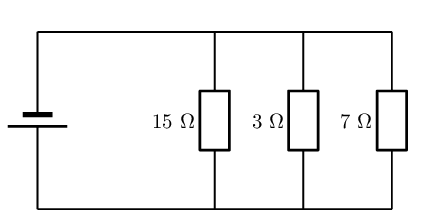What is the total resistance in the circuit?

### Analyse the question

We are told that the resistors in the circuit are in parallel circuit and that we need to calculate the total resistance. The value of the additional resistor has been given in the correct units, Ω.

### Apply the relevant principles

The total resistance for resistors in parallel has been given as

$\frac{1}{{R}_{P}} = \frac{1}{{R}_{1}} + \frac{1}{{R}_{2}} + \ldots$

We have three resistors and we now the resistances. In this case we have that:

\begin{align*} \frac{1}{{R}_{P}} & = \frac{1}{{R}_{1}} + \frac{1}{{R}_{2}} + \ldots \\ & \text{there are three resistors} \\ \frac{1}{{R}_{P}} & = \frac{1}{{R}_{1}} + \frac{1}{{R}_{2}} + \frac{1}{{R}_{3}} \\ & \text{add the fractions} \\ \frac{1}{{R}_{P}} & = \frac{1}{{R}_{1}} \times \frac{{R}_{2}{R}_{3}}{{R}_{2}{R}_{3}} + \frac{1}{{R}_{2}} \times \frac{{R}_{1}{R}_{3}}{{R}_{1}{R}_{3}} + \frac{1}{{R}_{3}} \times \frac{{R}_{1}{R}_{2}}{{R}_{1}{R}_{2}} \\ \frac{1}{{R}_{P}} & = \frac{{R}_{2}{R}_{3}}{{R}_{1}{R}_{2}{R}_{3}} + \frac{{R}_{1}{R}_{3}}{{R}_{1}{R}_{2}{R}_{3}} + \frac{{R}_{1}{R}_{2}}{{R}_{1}{R}_{2}{R}_{3}} \\ & \text{rearrange} \\ \frac{1}{{R}_{P}} & = \frac{{R}_{2}{R}_{3} + {R}_{1}{R}_{3} + {R}_{2}{R}_{3}}{{R}_{1}{R}_{2}{R}_{3}} \\ {R}_{P} & = \frac{{R}_{1}{R}_{2}{R}_{3}}{{R}_{2}{R}_{3} + {R}_{1}{R}_{3} + {R}_{2}{R}_{3}} \\ {R}_{P} & = \frac{\left(\text{15}\text{ Ω} \right)\left(\text{7}\text{ Ω} \right)\left(\text{3}\text{ Ω} \right)}{\left(\text{7}\text{ Ω} \right)\left(\text{3}\text{ Ω} \right) + \left(\text{15}\text{ Ω} \right)\left(\text{3}\text{ Ω}\right) + \left(\text{7}\text{ Ω} \right)\left(\text{15}\text{ Ω}\right)} \\ {R}_{P} & = \frac{315 {\Omega }^{3}}{21 {\Omega }^{2} + 45 {\Omega }^{2} + 105 {\Omega }^{2}} \\ {R}_{P} & = \frac{315 {\Omega }^{3}}{171 {\Omega }^{2}} \\ {R}_{P} & = \text{1,84}\text{ Ω} \end{align*}

### Quote the final result

The total resistance of the resistors in parallel is $$\text{1,84}$$ $$\text{Ω}$$

When calculating the resistance for complex resistor configurations, you can start with any combination of two resistors (in series or parallel) and calculate their total resistance. Then you can replace them with a single resistor that has the total resistance you calculated. Now use this new resistor in combination with any other resistor and repeat the process until there is only one resistor left. In the above example we could just have used the answer from the first example in parallel with the new resistor and we would get the same answer.

## Worked example 13: Parallel resistors III

We add a third parallel resistor to the first parallel worked example configuration (setup). The additional resistor has a resistance of $$\text{3}$$ $$\text{Ω}$$What is the total resistance in the circuit?

### Analyse the question

We are told that the resistors in the circuit are in parallel circuit and that we need to calculate the total resistance. The value of the additional resistor has been given in the correct units, Ω.

### Apply the relevant principles

We can swap the resistors around without changing the circuit:We have already calculated the total resistance of the two resistors in the dashed box to be $$\text{4,77}$$ $$\text{Ω}$$. We can replace these two resistors with a single resistor of $$\text{4,77}$$ $$\text{Ω}$$ to get:### Calculate the total resistance for the next pair of resistors

Then we use the formula for two parallel resistors again to get the total resistance for this new circuit:

\begin{align*} {R}_{P} & = \frac{{R}_{1}{R}_{2}}{{R}_{1}+{R}_{2}} \\ & = \frac{\left(\text{4,77}\text{ Ω} \right)\left(\text{3}\text{ Ω}\right)}{\text{4,77}\text{ Ω} + \text{3}\text{ Ω} } \\ & = \frac{\text{14,31} {\Omega }^{2}}{\text{11,77} \Omega } \\ & = \text{1,84}\text{ Ω} \end{align*}

### Quote the final result

The total resistance of the resistors in parallel is $$\text{1,84}$$ $$\text{Ω}$$. This is the same result as when we added all three resistors together at once.

## Resistance

Textbook Exercise 17.1

What is the unit of resistance called and what is its symbol?

Solution not yet available

Explain what happens to the total resistance of a circuit when resistors are added in series?

Solution not yet available

Explain what happens to the total resistance of a circuit when resistors are added in parallel?

Solution not yet available

Why do batteries go flat?

Solution not yet available